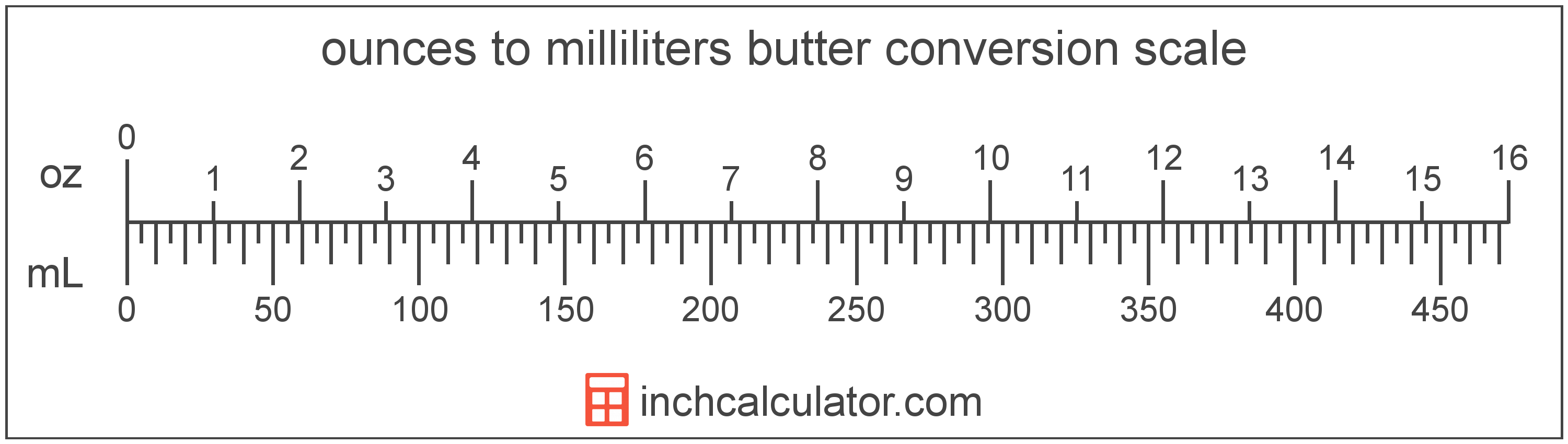# Convert Milliliters of Butter to Ounces

Enter the butter in milliliters below to get the value converted to ounces.

Results in Ounces:1 mL = 0.033814 oz

Do you want to convert ounces to milliliters?

## How to Convert Milliliters to Ounces

To convert a measurement in milliliters to a measurement in ounces, multiply the butter by the following conversion ratio: 0.033814 ounces/milliliter.

Since one milliliter of butter is equal to 0.033814 ounces, you can use this simple formula to convert:

ounces = milliliters × 0.033814

The butter in ounces is equal to the butter in milliliters multiplied by 0.033814.

For example, here's how to convert 5 milliliters to ounces using the formula above.
ounces = (5 mL × 0.033814) = 0.16907 oz## What is a Milliliter?

Butter is sometimes measured by volume in milliliters. One stick of butter contains about 118.3 milliliters.

The milliliter is an SI unit of volume in the metric system. A milliliter is sometimes also referred to as a millilitre. Milliliters can be abbreviated as mL; for example, 1 milliliter can be written as 1 mL.

## What is an Ounce?

In the US butter is sold in 16 ounce packages, which contain 1 pound of butter.

The ounce is a US customary and imperial unit of weight. Ounces can be abbreviated as oz; for example, 1 ounce can be written as 1 oz.

## Milliliter to Ounce Conversion Table

Table showing various milliliter measurements converted to ounces.
Milliliters Ounces
1 mL 0.033814 oz
2 mL 0.067628 oz
3 mL 0.101442 oz
4 mL 0.135256 oz
5 mL 0.16907 oz
6 mL 0.202884 oz
7 mL 0.236698 oz
8 mL 0.270512 oz
9 mL 0.304326 oz
10 mL 0.33814 oz
11 mL 0.371954 oz
12 mL 0.405768 oz
13 mL 0.439582 oz
14 mL 0.473396 oz
15 mL 0.50721 oz
16 mL 0.541024 oz
17 mL 0.574838 oz
18 mL 0.608652 oz
19 mL 0.642466 oz
20 mL 0.67628 oz
21 mL 0.710094 oz
22 mL 0.743908 oz
23 mL 0.777723 oz
24 mL 0.811537 oz
25 mL 0.845351 oz
26 mL 0.879165 oz
27 mL 0.912979 oz
28 mL 0.946793 oz
29 mL 0.980607 oz
30 mL 1.0144 oz
31 mL 1.0482 oz
32 mL 1.082 oz
33 mL 1.1159 oz
34 mL 1.1497 oz
35 mL 1.1835 oz
36 mL 1.2173 oz
37 mL 1.2511 oz
38 mL 1.2849 oz
39 mL 1.3187 oz
40 mL 1.3526 oz